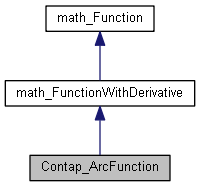# Contap_ArcFunction Class Reference

`#include <Contap_ArcFunction.hxx>`

Inheritance diagram for Contap_ArcFunction:[legend]

## Public Member Functions

Contap_ArcFunction ()

void Set (const Handle< Adaptor3d_HSurface > &S)

void Set (const gp_Dir &Direction)

void Set (const gp_Dir &Direction, const Standard_Real Angle)

void Set (const gp_Pnt &Eye)

void Set (const gp_Pnt &Eye, const Standard_Real Angle)

void Set (const Handle< Adaptor2d_HCurve2d > &A)

Standard_Boolean Value (const Standard_Real X, Standard_Real &F) override
Computes the value <F>of the function for the variable <X>. Returns True if the calculation were successfully done, False otherwise. More...

Standard_Boolean Derivative (const Standard_Real X, Standard_Real &D) override
Computes the derivative <D> of the function for the variable <X>. Returns True if the calculation were successfully done, False otherwise. More...

Standard_Boolean Values (const Standard_Real X, Standard_Real &F, Standard_Real &D) override
Computes the value <F> and the derivative <D> of the function for the variable <X>. Returns True if the calculation were successfully done, False otherwise. More...

Standard_Integer NbSamples () const

virtual Standard_Integer GetStateNumber () override
returns the state of the function corresponding to the latest call of any methods associated with the function. This function is called by each of the algorithms described later which defined the function Integer Algorithm::StateNumber(). The algorithm has the responsibility to call this function when it has found a solution (i.e. a root or a minimum) and has to maintain the association between the solution found and this StateNumber. Byu default, this method returns 0 (which means for the algorithm: no state has been saved). It is the responsibility of the programmer to decide if he needs to save the current state of the function and to return an Integer that allows retrieval of the state. More...

const gp_PntValpoint (const Standard_Integer Index) constPublic Member Functions inherited from math_FunctionWithDerivative
virtual ~math_FunctionWithDerivative ()Public Member Functions inherited from math_Function
virtual ~math_Function ()
Virtual destructor, for safe inheritance. More...

## Constructor & Destructor Documentation

 Contap_ArcFunction::Contap_ArcFunction ( )

## Member Function Documentation

 Standard_Boolean Contap_ArcFunction::Derivative ( const Standard_Real X, Standard_Real & D )
overridevirtual

Computes the derivative <D> of the function for the variable <X>. Returns True if the calculation were successfully done, False otherwise.

Implements math_FunctionWithDerivative.

 virtual Standard_Integer Contap_ArcFunction::GetStateNumber ( )
overridevirtual

returns the state of the function corresponding to the latest call of any methods associated with the function. This function is called by each of the algorithms described later which defined the function Integer Algorithm::StateNumber(). The algorithm has the responsibility to call this function when it has found a solution (i.e. a root or a minimum) and has to maintain the association between the solution found and this StateNumber. Byu default, this method returns 0 (which means for the algorithm: no state has been saved). It is the responsibility of the programmer to decide if he needs to save the current state of the function and to return an Integer that allows retrieval of the state.

Reimplemented from math_Function.

 Standard_Integer Contap_ArcFunction::NbSamples ( ) const
 void Contap_ArcFunction::Set ( const Handle< Adaptor3d_HSurface > & S )
 void Contap_ArcFunction::Set ( const gp_Dir & Direction )
 void Contap_ArcFunction::Set ( const gp_Dir & Direction, const Standard_Real Angle )
 void Contap_ArcFunction::Set ( const gp_Pnt & Eye )
 void Contap_ArcFunction::Set ( const gp_Pnt & Eye, const Standard_Real Angle )
 void Contap_ArcFunction::Set ( const Handle< Adaptor2d_HCurve2d > & A )
 const gp_Pnt& Contap_ArcFunction::Valpoint ( const Standard_Integer Index ) const
 Standard_Boolean Contap_ArcFunction::Value ( const Standard_Real X, Standard_Real & F )
overridevirtual

Computes the value <F>of the function for the variable <X>. Returns True if the calculation were successfully done, False otherwise.

Implements math_FunctionWithDerivative.

 Standard_Boolean Contap_ArcFunction::Values ( const Standard_Real X, Standard_Real & F, Standard_Real & D )
overridevirtual

Computes the value <F> and the derivative <D> of the function for the variable <X>. Returns True if the calculation were successfully done, False otherwise.

Implements math_FunctionWithDerivative.

The documentation for this class was generated from the following file: